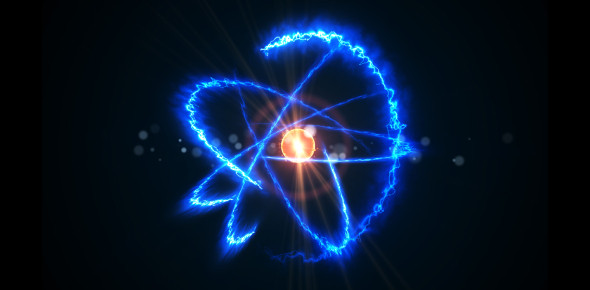# Physics Quiz: Basic Exam MCQ!

10 Questions | Attempts: 101Settings.

• 1.
Product of Mass and Velocity.
• A.

Momentum

• B.

Power

• C.

Impulse

• D.

Energy

• 2.
The change in velocity per unit time.
• A.

Speed

• B.

Distance

• C.

Acceleration

• D.

Height

• 3.
The product of force and time and equal to the change in momentum.
• A.

Impulse

• B.

Collision

• C.

Momentum

• D.

Power

• 4.
The two identical cars are traveling along EDSA. Which of the two cars have greater momentum?
• A.

The faster car

• B.

Neither of the two cars

• C.

The slower car

• D.

Momentum cannot be determined

• 5.
A bus has an initial velocity of 30 m/s. If its final velocity is 40 m/s, what is the average velocity?
• A.

20 m/s

• B.

25m/s

• C.

30 m/s

• D.

35 m/s

• 6.
Acceleration is a change in velocity per unit time. In what conditions, acceleration is zero?
• A.

No change in direction but there is a change in velocity

• B.

No change in both direction and velocity

• C.

No change in velocity but there is a change in direction

• D.

No change in impulse and momentum

• 7.
Which factors show that momentum is possible only if these are present?
• A.

Mass and force

• B.

Mass and velocity

• C.

Mass and acceleration

• D.

Mass and time

• 8.
Kinetic energy may be lost during a collision when it is converted to heat and other forms of energy. When the total kinetic energy of the system does not change, and colliding objects bounce off after the collision, it is said to be:
• A.

Elastic

• B.

Inelastic

• C.

Neither A nor C

• D.

Both A and C

• 9.
The harmful effects on the environment of the heat exhausted by heat engines and the Refrigerator into the environment which is a consequence of low thermal efficiency are known:
• A.

Heat

• B.

Work

• C.

Thermal pollution

• D.

Combustion

• 10.
What energy transformation takes place in a refrigerator?
• A.

Electrical to mechanical

• B.

Heat to mechanical

• C.

Mechanical to electrical

• D.

Chemical to mechanical

## Related TopicsBack to top
×

Wait!
Here's an interesting quiz for you.